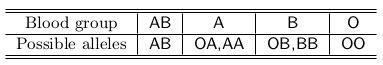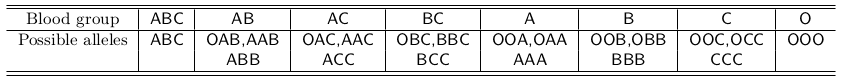### B - Blood groups

##### Languages: C, C++, Java, JavaScript, ... (details)

There are four possible blood groups for humans: $\texttt{AB}$, $\texttt{A}$, $\texttt{B}$ and $\texttt{O}$, meaning that the red blood cells have antigens of types, respectively, $\texttt{A}$ and $\texttt{B}$, only $\texttt{A}$, only $\texttt{B}$, and no antigen at all. Our blood group is determined by two alleles in our DNA. Each allele is of type either $\texttt{A}$, $\texttt{B}$ or $\texttt{O}$. The following table lists the possible allele combinations someone may have for each blood group:We inherit exactly one allele from each of our two parents. So, given the blood groups of the two parents, we can say for sure if some blood group is possible, or not, in their offspring. For example, if the blood groups of the two parents are $\texttt{AB}$ and $\texttt{B}$, then the possible allele combinations for them are, respectively, {$\texttt{AB}$} and {$\texttt{OB}$,$\texttt{BB}$}. Since the order of the alleles does not matter, the possible allele combinations for the offspring are {$\texttt{OA}$,$\texttt{AB}$,$\texttt{OB}$,$\texttt{BB}$}. That means the blood groups $\texttt{AB}$, $\texttt{A}$ and $\texttt{B}$ are possible in their offspring, but the blood group $\texttt{O}$ is not. Very nice indeed! But what if life on Earth had evolved so that a person had three parents, three alleles, and three different antigen types? The allele combinations would look like this:If the blood groups of the three parents are $\texttt{A}$, $\texttt{BC}$ and $\texttt{O}$, then all blood groups are possible in their offspring, except groups $\texttt{BC}$ and $\texttt{ABC}$. The universe is vast! There may be, out there in space, some form of life whose individuals have $N$ parents, $N$ alleles, and $N$ different antigen types. Given the blood groups for the $N$ parents, and a list of $Q$ blood groups to test, your program has to determine which ones are possible, and which ones are not, in the offspring of the given parents.

#### Input

The first line contains two integers $N$ and $Q$, representing respectively the number of parents (and alleles, and antigen types) and the number of queries ($1 \leq N \leq 100$ and $1 \leq Q \leq 40$). Each of the next $N$ lines describes the blood group of a parent. After that, each of the next $Q$ lines describes a blood group to test. Antigen types are identified with distinct integers from $1$ to $N$, not letters. Each line describing a blood group contains an integer $B$ indicating the number of antigen types in the blood group ($0 \leq B \leq N$), followed by $B$ different integers $C_1, C_2, ..., C_B$ representing the antigen types present in the blood group ($1 \leq C_i \leq N$ for $i = 1, 2, ... , B$).

#### Output

For each of the $Q$ queries, output a line with the uppercase letter $\texttt{Y}$ if the corresponding blood group is possible in the offspring of the given parents; otherwise output the uppercase letter $\texttt{N}$. Write the results in the same order that the queries appear in the input.

#### Sample test(s)

Input
2 1 2 2 1 1 2 0
Output
N
Input
3 4 1 1 2 2 3 0 1 3 3 2 1 3 2 1 2 2 3 2
Output
Y N Y N
Input
4 3 4 2 1 3 4 4 2 1 3 4 1 1 1 2 1 3 2 2 1 0
Output
Y Y N